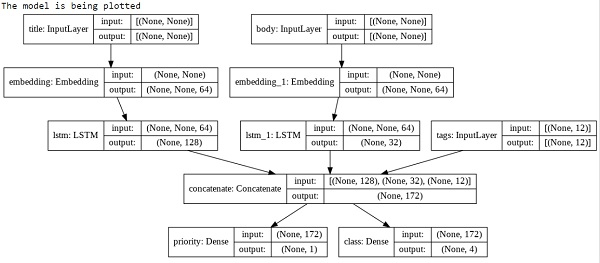# How can Keras be used to plot the model using Python?

Tensorflow is a machine learning framework that is provided by Google. It is an open-source framework used in conjunction with Python to implement algorithms, deep learning applications and much more. It is used in research and for production purposes. It has optimization techniques that help in performing complicated mathematical operations quickly. Tensor is a data structure used in TensorFlow. It helps connect edges in a flow diagram. This flow diagram is known as the ‘Data flow graph’. Tensors are nothing but multidimensional array or a list.

Keras was developed as a part of research for the project ONEIROS (Open ended Neuro-Electronic Intelligent Robot Operating System). Keras is a deep learning API, which is written in Python. It is a high-level API that has a productive interface that helps solve machine learning problems. It runs on top of Tensorflow framework. It was built to help experiment in a quick manner. It provides essential abstractions and building blocks that are essential in developing and encapsulating machine learning solutions.

Keras is already present within the Tensorflow package. It can be accessed using the below line of code.

import tensorflow
from tensorflow import keras

The Keras functional API helps create models that are more flexible in comparison to models created using sequential API. The functional API can work with models that have non-linear topology, can share layers and work with multiple inputs and outputs. A deep learning model is usually a directed acyclic graph (DAG) that contains multiple layers. The functional API helps build the graph of layers.

We are using the Google Colaboratory to run the below code. Google Colab or Colaboratory helps run Python code over the browser and requires zero configuration and free access to GPUs (Graphical Processing Units). Colaboratory has been built on top of Jupyter Notebook. Following is the code snippet to plot the model with Keras −

## Example

print("The model is being plotted")
keras.utils.plot_model(model, "multi_model.png", show_shapes=True)

Code credit − https://www.tensorflow.org/guide/keras/functional

## Output## Explanation

• The model can be plotted as a graph-of-layers.

• This can be achieved using the ‘plot_model’ method.